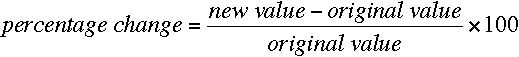SEARCH HOMEMath Central Quandaries & QueriesQuestion from Bill: How to calculate the Percentage Increase or Decrease of a $value. Example - Site Spend for 01-01-2008 thru 07-31-08 was$ 3,465,660 Site Spend, 2009 YTD as of 07-31-09, was $1,673,405 Site Spend down what % between these two amounts ?Bill, If some value changes over time then the percentage change is the change as a percentage of the original value. That isIn your case the change is new value - original value =$1,673,405 - $3,465,660 = -$1,792,255. Hence the percentage change is ( - $1,792,255/$3,465,660) × 100 = -51.7%. Since this value is negative there has been a decrease so you might say there has been a 51.7% decrease.

I hope this helps,
PennyMath Central is supported by the University of Regina and The Pacific Institute for the Mathematical Sciences.